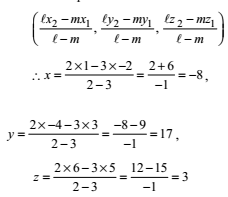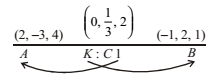## NCERT Solutions for Class 11 Maths Chapter 12 Introduction to Three Dimensional Geometry Exercise 12.3

Chapter 12 Introduction to Three Dimensional Geometry Exercise 12.3 NCERT Solutions for Class 11 Maths are helpful for the purpose of examinations. You can clear your basic doubts by practicing these questions. Class 11 Maths NCERT Solutions prepared by Studyrankers experts that are correct and detailed so you can use them whenever you find a problem difficult.1. Find the co ordinates of the point which divides the line segment joining the points (–2, 3, 5) and (1, – 4, 6) in the ratio (i) 2: 3 internally (ii) 2: 3 externally.

(i) Let P(x, y, z) be the point which divides the line segment joining the points (–2, 3, 5) and (1, –4, 6) in the ratio 2: 3 internally. Coordinates of point P are given by ratio (l, m)(ii) If P divides AB externally in the ratio l: m then the point P is∴The point (–8, 17, 3) divides AB externally in the ratio 2: 3.

2. Given that P(3, 2, –4), Q(5, 4, –6) and R(9, 8, –10) are collinear. Find the ratio in which Q divides PR.

Let Q be divide PR in the ratio k: 1This shows that the point Q lies on it and it divides PR in the ratio 1: 2.

3. Find the ratio in which the YZ-plane divide the line segment formed by joining the points (– 2, 4, 7) and (3, – 5, 8).

Let the points be A(–2, 4, 7) and B(3, –5, 8) on yz-plane, x-coordinate = 0.Let the ratio be K: 1.
The coordinates of C areHence required ratio is 2: 3.

4. Using Section Formula, show that the points A(2, – 3, 4), B(–1, 2, 1) and C(0, 1/3 , 2) are collinear.

Given A(2, –3, 4), B(–1, 2, 1) and C(0, 1/3, 2) let C divides AB in the ratio K: 1.Now coordinates of C are
((-k + 2)/(k + 1)) , ((2k – 3)/(k + 1)) , ((k + 4)/(k + 1))
Let (-k + 2)/(k + 1) = 0 => k = 2
Again (2k – 3)/(k + 1) = (2 × 2 – 3)/(2 + 1) = 1/3
And (k + 4)/(k + 1) = (2 + 4)/(2 + 1) = 2
Thus the point C(0, 1/3 , 2) divides AB in the ratio 2: 1 and is the same as C.

5. Find the coordinates of the points which trisect the line segment joining the points P(4, 2, – 6) and Q(10, – 16, 6).